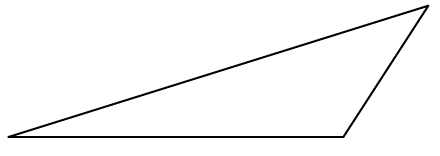### Home > GC > Chapter 3 > Lesson 3.1.1 > Problem3-7

3-7.Examine the triangle at right.

Think of how each angle of the triangle relates to a $90º$ angle.

1. Estimate the measure of each angle of the triangle above.

2. Given only its shape, what is the best name for this triangle?